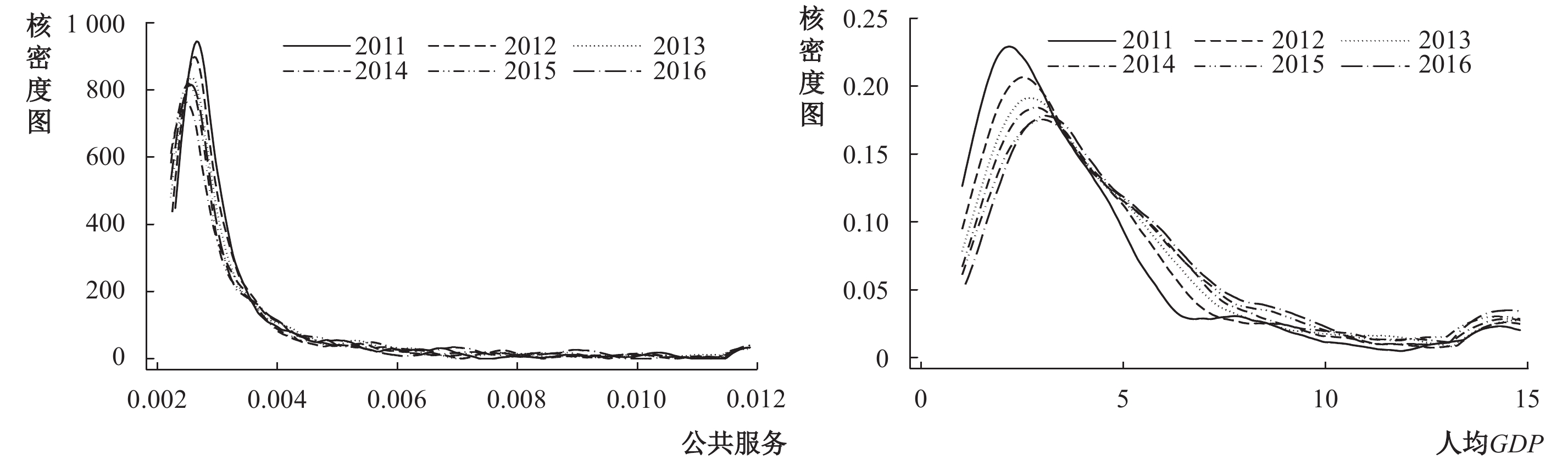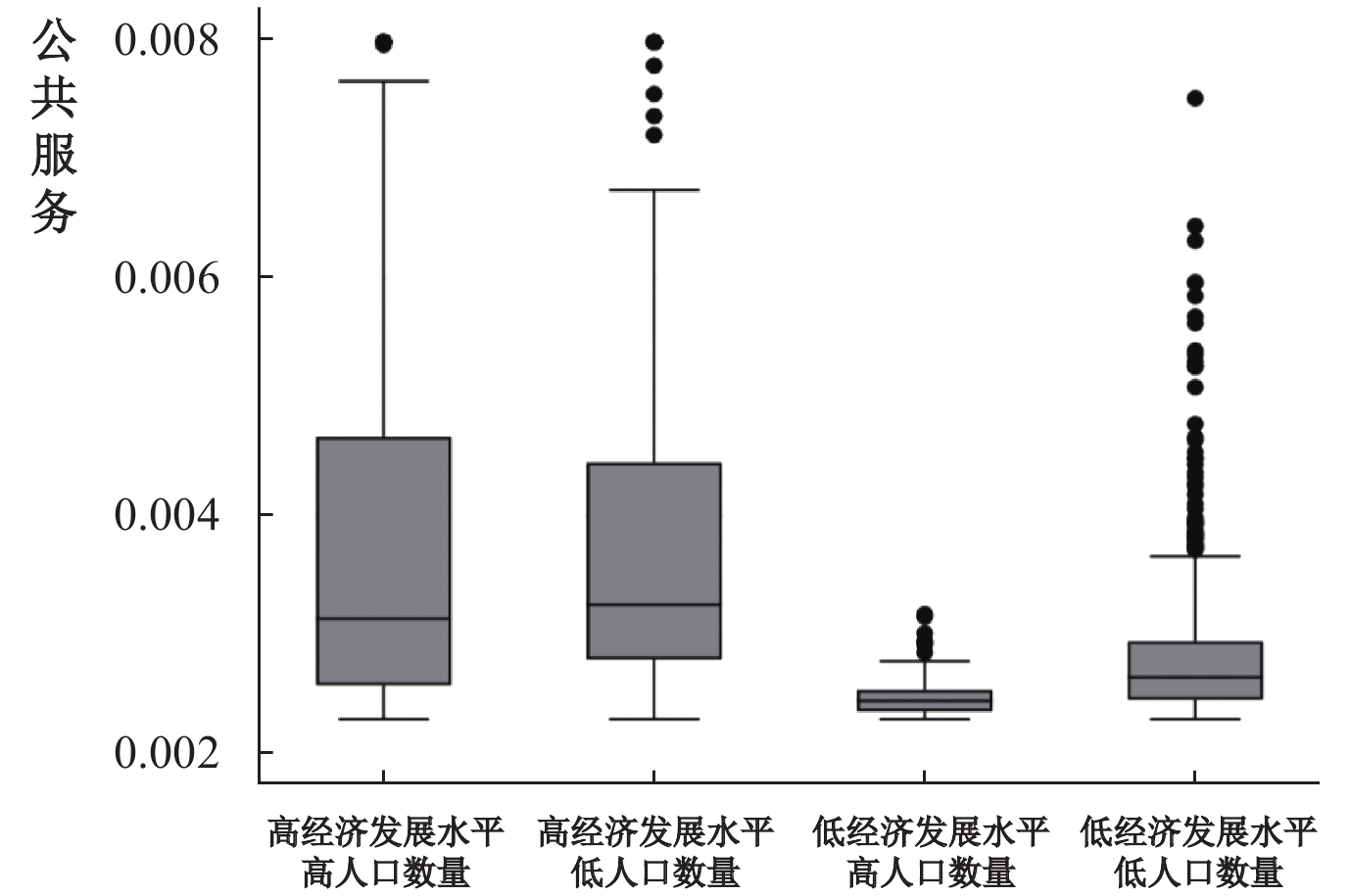﻿ 公共服务竞争、要素流动与区域经济新格局Deng Huihui, Xue Yi, Yang Luxin. Public service competition，factor flow and new regional economic pattern[J]. Journal of Finance and Economics, 2021, 47(8): 34-48.1. 对外经济贸易大学 国际经济研究院，北京 100029;
2. 对外经济贸易大学 国际经济贸易学院，北京 100029

Public Service Competition，Factor Flow and New Regional Economic Pattern
Deng Huihui1, Xue Yi2, Yang Luxin1
1. Institute of International Economy，University of International Business and Economics，Beijing 100029，China;
2. School of International Trade and Economics，University of International Business and Economics，Beijing 100029，China
Summary: In the past 40 years, local government competition has played a very important role in China’s economic growth. However, the rapid economic growth has been accompanied by challenges in public health, public education, resources and the environment, etc. How to improve the level of public services and gather innovative elements such as human and capital with a better capacity of carrying is a realistic problem that urgently needs to be considered. The outbreak of COVID-19 epidemic is a major test for the national and local governance system. While forcing the improvement of local public service capabilities, the competition for public services around the prevention and control of the epidemic becomes visible. To clarify the realistic background and institutional constraints that lead local competition to economic growth rather than public services, and to guide public services to become a new competition yardstick for local governments through new institutional arrangements, can provide new impetus for China’s regional coordinated development, which is also the internal requirement of the modernization of national governance for local government reform. Firstly, this paper adopts a two-region model to show that the failure of local public services to coordinate with economic growth is a natural result of local government behavior choices. Under the new incentive and restraint mechanism, it is possible to move from economic growth competition to public service competition. Secondly, based on the typical facts of local public services, around the current major adjustments in China’s productivity layout, the empirical tests find that the full flow of population and capital elements in the new era relies on the spatial network system with infrastructure and public services as carriers, and public services have become a carrier for central cities and urban agglomerations to attract core resources. Furthermore, from the central-local-social perspective, based on a two-regime spatial Durbin model with fixed effects, the results show that reforming the performance evaluation index system, expanding local government information disclosure, enhancing public opinion supervision and public participation, and stimulating the vitality of social organizations provide a realistic and feasible way to promote the establishment of a competitive relationship of “racing to the top” and “aiming high” to improve the overall public service level and promote the equalization of public services.
Key words: public services    local government competition    factor flow    regional coordinated development图 1 公共服务水平与经济发展水平的核密度图

(一) 制度背景

(二) 理论假说

$U$
）如下：

 $U=Y+\alpha S$ (1)
 $Y={h}_{1}{h}_{2}+{h}_{1}+\varepsilon$ (2)

$\alpha$

${h}_{1}$
）来决定，同时地方政府为公共服务水平提升而做出的努力（
${h}_{2}$
）也能转化为经济增长的动力，比如较高的政府办事效率、社会福利等都是吸引企业入驻和人才迁入的条件。由于公共服务水平由地方政府的努力程度决定，这里令
$S={h}_{2}$
，此时，居民的效用函数可转化为：

 $U={h}_{1}{h}_{2}+{h}_{1}+\alpha {h}_{2}+\varepsilon$ (3)

 $\begin{array}{l} \;\;\;\;\;\;\;\;\;P=Prob\left({Y}^{i}>{Y}^{j}\right)=Prob\left({h}_{1}^{i}{h}_{2}^{i}+{h}_{1}^{i}+{\varepsilon }^{i}>{h}_{1}^{j}{h}_{2}^{j}+{h}_{1}^{j}+{\varepsilon }^{j}\right) \\ =\displaystyle{\int }_{-\infty }^{+\infty }{\int }_{-\infty }^{{h}_{1}^{i}{h}_{2}^{i}+{h}_{1}^{i}-{h}_{1}^{j}{h}_{2}^{j}+{h}_{1}^{j}+{\varepsilon }^{i}}\dfrac{{e}^{-1/2[{\left({\varepsilon }^{i}\right)}^{2}/{\sigma }_{1}^{2}]}}{{\sigma }_{1}\sqrt{2\pi }}\frac{{e}^{-1/2[{\left({\varepsilon }^{j}\right)}^{2}/{\sigma }_{1}^{2}]}}{{\sigma }_{1}\sqrt{2\pi }}d{\varepsilon }^{j}d{\varepsilon }^{i} \end{array}$ (4)

 $C=C\left({h}_{1},{h}_{2}\right)=1/\left[2\beta {({h}_{1}+{h}_{2})}^{2}\right]$ (5)

 ${\rm{ma}}{{\rm{x}}_{{h_1},{h_2}}}PW+\left(1-P\right)w-1/\left[2\beta {({h}_{1}+{h}_{2})}^{2}\right]$ (6)

$W$

$w$

 $\frac{\partial P}{\partial {h}_{1}}W-\frac{\partial P}{\partial {h}_{1}}w-\beta \left({h}_{1}+{h}_{2}\right)=0$ (7)
 $\frac{\partial P}{\partial {h}_{2}}W-\frac{\partial P}{\partial {h}_{2}}w-\beta \left({h}_{1}+{h}_{2}\right)=0$ (8)

${h}_{1}^{i}$
${h}_{2}^{i}$

 $\frac{1+{\widehat{h}}_{2}}{2{\sigma }_{1}\sqrt{\pi }}\left(W-w\right)= \beta \left({\widehat{h}}_{1}+{\widehat{h}}_{2}\right)$ (9)
 $\frac{{\widehat{h}}_{1}}{2{\sigma }_{1}\sqrt{\pi }}\left(W-w\right)= \beta \left({\widehat{h}}_{1}+{\widehat{h}}_{2}\right)$ (10)

 ${\widehat{h}}_{2}=\dfrac{\dfrac{2{\sigma }_{1}\sqrt{\pi }\beta }{(W-w)}-1}{1-\dfrac{4{\sigma }_{1}\sqrt{\pi }\beta }{(W-w)}}$ (11)
 ${\widehat{h}}_{1}=1+\dfrac{\dfrac{2{\sigma }_{1}\sqrt{\pi }\beta }{(W-w)}-1}{1-\dfrac{4{\sigma }_{1}\sqrt{\pi }\beta }{(W-w)}}$ (12)

$\theta =\dfrac{2{\sigma }_{1}\sqrt{\pi }\beta }{(W-w)}$
，为保证地方政府的努力程度不为小于零的数值，不难看出
$0.5<\theta <1$
，基于这一条件可得到
${\widehat{h}}_{1}>{\widehat{h}}_{2}$
。而当
$\theta >1$

${\widehat{h}}_{2}=0,{\widehat{h}}_{1}>0$
，即在以GDP为主的晋升考核机制下，地方政府“为增长而竞争”的行为不利于公共服务水平的提高。原因在于招商引资、举债投资等方式能使地方政府中获得更大的晋升机会，而难以产生快速经济效益的科教文卫等公共服务部门的支出必然受到抑制，使财政支出结构产生偏向（尹恒和朱虹，2011），不利于公共服务水平的提高以及基本公共服务均等化。要调动地方官员的努力，就必须建立明确的考核机制，而明确的考核机制依赖于有效的评价体系。基于此，本文提出假说1：

 ${U}^{‘}=U+\delta ={h}_{1}{h}_{2}+{h}_{1}+\alpha {h}_{2}+\varepsilon +\delta$ (13)

$\delta$

$\delta >0$

 $\begin{array}{*{20}{c}} { P=Prob\left({{U}^{‘}}^{i}>{{U}^{‘}}^{j}\right)=Prob\left({h}_{1}^{i}{h}_{2}^{i}+{h}_{1}^{i}+\alpha {h}_{2}^{i}+{\varepsilon }^{i}+{\delta }^{i}>{h}_{1}^{j}{h}_{2}^{j}+{h}_{1}^{j}+\alpha {h}_{2}^{j}+{\varepsilon }^{j}+{\delta }^{j}\right)}\\ =\\ \smallint _{ - \infty }^{ + \infty }\smallint _{ - \infty }^{ + \infty }\smallint _{ - \infty }^{ + \infty }{\int }_{-\infty }^{{h}_{1}^{i}{h}_{2}^{i}+{h}_{1}^{i}+\alpha {h}_{2}^{i}-{h}_{1}^{j}{h}_{2}^{j}-{h}_{1}^{j}-\alpha {h}_{2}^{j}+{\varepsilon }^{i}+{\delta }^{i}-{\delta }^{j}}\dfrac{{e}^{-\frac{{({\varepsilon }^{i})}^{2}+{({\varepsilon }^{j})}^{2}}{2{\sigma }_{1}^{2}}}}{2\pi {\sigma }_{1}^{2}}\dfrac{{e}^{-\frac{{({\delta }^{i})}^{2}+{({\delta }^{j})}^{2}}{2{\sigma }_{2}^{2}}}}{2\pi {\sigma }_{2}^{2}}d{\varepsilon }^{j}d{\varepsilon }^{i}d{\delta }^{i}d{\delta }^{j} } \end{array}$ (14)

${h}_{1}^{i}$
${h}_{2}^{i}$

 $\frac{1+{\widetilde {h}}_{2}}{2\sqrt{{\sigma }_{1}^{2}+{\sigma }_{2}^{2}}\sqrt{\pi }}\left(W-w\right)= \beta \left({\widetilde {h}}_{1}+{\widetilde {h}}_{2}\right)$ (15)
 $\frac{\alpha +{\widetilde {h}}_{1}}{2\sqrt{{\sigma }_{1}^{2}+{\sigma }_{2}^{2}}\sqrt{\pi }}\left(W-w\right)= \beta \left({\widetilde {h}}_{1}+{\widetilde {h}}_{2}\right)$ (16)

 ${\widetilde {h}}_{1}=\dfrac{\dfrac{2\left(\alpha -1\right)\sqrt{{\sigma }_{1}^{2}+{\sigma }_{2}^{2}}\sqrt{\pi }\beta }{(W-w)}-\alpha }{1-\dfrac{4\sqrt{{\sigma }_{1}^{2}+{\sigma }_{2}^{2}}\sqrt{\pi }\beta }{(W-w)}}$ (17)
 ${\widetilde {h}}_{2}=\dfrac{\dfrac{2\left(\alpha -1\right)\sqrt{{\sigma }_{1}^{2}+{\sigma }_{2}^{2}}\sqrt{\pi }\beta }{(W-w)}-\alpha }{1-\dfrac{4\sqrt{{\sigma }_{1}^{2}+{\sigma }_{2}^{2}}\sqrt{\pi }\beta }{(W-w)}}+\alpha -1$ (18)

$\mu =\dfrac{2\sqrt{{\sigma }_{1}^{2}+{\sigma }_{2}^{2}}\sqrt{\pi }\beta }{(W-w)}$
，公式（17）—（18）可转化为：

 ${\widetilde {h}}_{1}=\frac{\alpha +1}{2-1/\mu }-\alpha$ (19)
 ${\widetilde {h}}_{2}=\frac{\alpha +1}{2-1/\mu }-1$ (20)

$\alpha <1$
$\mu \leqslant \dfrac{1}{1-\alpha }$

${\widetilde {h}}_{1}>0$
${\widetilde {h}}_{2}=0$
；而当
$\alpha >1$
$\mu \leqslant \dfrac{\alpha }{\alpha -1}$

${\widetilde {h}}_{1}=0$
${\widetilde {h}}_{2}>0$
。这一结果表明居民对公共服务水平的偏好（
$\alpha$
）越强，地方政府越愿意为公共服务水平的提高做出努力。改革开放40多年来，中国的生产力显著提高，公众对于美好生活的追求更加多元化，如更好的教育、更高的医疗水平等这些集中体现在公共领域的需求。因此，假定居民对公共服务水平的偏好为
$\alpha >1$

$\mu$

${\sigma }_{1}$
${\sigma }_{2}$

${\sigma }_{1}$

${\sigma }_{2}$

${\widetilde {h}}_{2}$

2019年8月，中央财经委员会第五次会议聚焦优势互补、高质量发展的区域经济布局，强调围绕当前中国生产力布局的重大调整，让中心城市和城市群成为承载发展要素的主要空间形式。可以预见，未来中国的经济和人口将进一步向中心城市以及中心城市周边的都市圈、城市群等空间集中，与此同时，驱动区域经济发展格局的路径和要素空间转移的逻辑也在调整。改革开放前40年要素流动逻辑是以工业园区建设为主导的人跟着产业走，那么在新时期要素的流动逻辑是什么呢？本节进行验证。

(一) 公共服务指标体系

 一级指标 二级指标 三级指标 公共服务（ $SERVI$ ） 公共教育（EDU） 人均教育经费a1 高等学校平均在校生数人a2 普通高校生师比a3 科技事业（TECH） 人均专利申请授权数b1 人均科技经费b2 人均发明专利数b3 公共卫生（HEALTH） 人均医疗卫生费投入c1 每万人拥有卫生技术人员数c2 每万人医疗机构床位数c3 社会保障（SOCIAL） 社会保障支出占财政支出比重d1 城镇医疗保险覆盖率d2 失业保险覆盖率d3 养老保险覆盖率d4 公共设施（UTILITY） 人均道路面积e1 建成区排水管道密度e2 城市燃气普及率e3 生态环境（ECOLOGY） 污水处理厂集中处理率f1 生活垃圾无害化处理率f2 建成区绿化覆盖率f3

 ${x}_{ik}^{'}=({x}_{ik}-{\overline {x}}_{j})/{sd}_{k}$ (21)
 ${x}_{ik}^{'}=({\overline {x}}_{j}-{x}_{ik})/{sd}_{k}$ (22)

${x}_{ik}^{'}$
i省份 k指标标准化后的数据，
${x}_{ik}$

${\overline {x}}_{j}$
${sd}_{k}$

${X}_{ik}$

${r}_{ik}$

 ${r}_{ik}={X}_{ik}/{\sum }_{i=1}^{n}{X}_{ik}$ (23)

${e}_{ik}$

 ${e}_{ik}=-\frac{1}{\ln \left(n\right)}{\sum }_{i=1}^{n}{r}_{ik}\ln \left({r}_{ik}\right)$ (24)

 ${w}_{ik}=(1-{e}_{ik})/{\sum }_{k=1}^{m}(1-{e}_{ik})$ (25)

 ${SERVI}_{i}={\sum }_{k=1}^{m}{w}_{ik}{r}_{ik}$ (26)图 2 公共服务与GDP、人口的关联

(二) 公共服务与要素流动

 ${MIGRAT}_{it}=\alpha +\beta {SERVI}_{it}+\theta {X}_{it}+{\varepsilon }_{it}$ (27)

${MIGRAT}_{it}$

${SERVI}_{it}$
${X}_{it}$

${\varepsilon }_{it}$

${SERVI}_{it}$

 （1） $MIGRAT$ （2） $CAPITAL$ （3）第一阶段估计 $MIGRAT$ （4）第二阶段估计 $CAPITAL$ $SERVI$ 5.4982* 1.5523* 0.1324** （1.77） （1.76） （2.01） $MIGRAT$ 0.4683* （1.72） 控制变量 控制 控制 控制 控制 地区效应 控制 控制 控制 控制 时间效应 控制 控制 控制 控制 Anderson canon. corr. LM statistic 3.302 * 3.302 * 10.186*** Cragg-Donald Wald F statistic 8.965 >15% IV size 8.965>15%IV size 5.108>15%IV size 注：***、**和*分别表示在1%、5%和10%的显著性水平上显著，括号内是相应的t值（下表同）。限于篇幅仅报告关键变量的估计结果，其他结果备索。

 ${CAPITAL}_{it}=\alpha +\beta {SERVI}_{it}+\theta {X}_{it}+{\varepsilon }_{it}$ (28)

${CAPITAL}_{it}$

${SERVI}_{it}$

 ${MIGRAT}_{it}=\alpha +\beta {SERVI}_{it}+\theta {X}_{it}+{\varepsilon }_{it}$ (29)
 ${CAPITAL}_{it}=\alpha +\omega {MIGRAT}_{it}+\theta {X}_{it}+{\varepsilon }_{it}$ (30)

 $\begin{array}{l} {FACTOR}_{it}={\delta }_{1}{\sum }_{j=1}^{N}{w}_{ij}{FACTOR}_{it}+{\delta }_{2}{SERVI}_{it}+{\delta }_{3}{\sum }_{j=1}^{N}{w}_{ij}{SERVI}_{it}+{\delta }_{4}{X}_{it}+{\mu }_{i}+{\lambda }_{t}+{\varepsilon }_{it} \end{array}$ (31)

${FACTOR}_{it}$

${MIGRAT}_{it}$
${CAPITAL}_{it}$
${\mu }_{i}$
${\lambda }_{t}$

 （1） $MIGRAT$ （2） $CAPITAL$ $SERVI$ 0.3638*** 0.0734*** （8.39） （5.21） $W\_SERVI$ 0.3159 −0.2186 （0.66） （−1.46） $RHO$ 0.1958 0.6985*** （1.38） （10.16） 控制变量 控制 控制 地区效应 控制 控制 时间效应 控制 控制

(一) 地区公共服务竞争格局

 $\begin{array}{l} {SERVI}_{it}={\rho }_{1}{D}_{it}\displaystyle\sum _{j=1}^{274}{w}_{ij}{SERVI}_{jt}+{\rho }_{2}\left(1-{D}_{it}\right)\sum _{j=1}^{274}{w}_{ij}{SERVI}_{jt}+\beta {X}_{it}+\alpha +{\mu }_{i}+{\lambda }_{t}+{\varepsilon }_{it} \end{array}$ (32)
 ${D}_{it,1}=\left\{\begin{array}{l}1,\;{SERVI}_{it}>\displaystyle\sum _{j=1}^{274}{w}_{ij}{SERVI}_{jt}\;\;{\text{其中}}i\ne j\\ 0,\;{\text{其他}}\end{array}\right.$ (33)

${SERVI}_{it}$

$i$

$t$

${D}_{it}$

${w}_{ij}$

$i\ne j$

$1/{d}^{2}$
；当
$i=j$

${X}_{it}$

$\alpha$

${\mu }_{i}$
${\lambda }_{t}$

${\varepsilon }_{it}$

${\rho }_{1}$
${\rho }_{2}$

${\rho }_{1}$

${\rho }_{2}$

${\rho }_{1}$
${\rho }_{2}$

 ${\rho }_{1}$ ${\rho }_{2}$ 竞争策略 ${\rho }_{1}$ ${\rho }_{2}$ 竞争策略 ${\rho }_{1}$ ${\rho }_{2}$ 竞争策略 正 正 逐顶竞争 不显著 正 遇弱则强 负 正 遇强则弱、遇弱则强 正 负 见贤思齐、见劣自缓 不显著 负 见劣自缓 负 负 逐底竞争 正 不显著 见贤思齐 不显著 不显著 无竞争 负 不显著 遇强则弱

“两区制”空间杜宾模型的具体估计结果见表5，限于篇幅，本文仅报告地区间公共服务的空间竞争情况（即

$\rho 1$
$\rho 2$
）。如表5的模型（1）所示，
$\rho 1$

$\rho 2$

$\rho 1$

$\rho 2$

$\rho 1$
$\rho 2$

 （1）公共服务 （2）公共教育 （3）科技事业 （4）公共卫生 （5）社会保障 （6）公共设施 （7）生态保护 $\rho 1$ 0.3089 −0.1816 0.2946 0.1555 0.1620 −0.3263 −1.2488*** （0.99） （−0.67） （0.93） （0.50） （0.54） （−1.08） （−4.02） $\rho 2$ −0.9789*** 0.3087 −1.0741*** −0.7989** −0.6495** −0.1689 0.0346 （−2.95） （1.08） （−3.20） （−2.40） （−2.03） （−0.52） （0.10） 控制变量 控制 控制 控制 控制 控制 控制 控制 地区效应 控制 控制 控制 控制 控制 控制 控制 时间效应 控制 控制 控制 控制 控制 控制 控制

(二) 推动地方公共服务良性竞争的路径选择

1. 构建新发展理念引领下的绩效考核体系

$\rho 1$

$\rho 2$

 （1）新政绩考核 （2）信息公开 （3）公众参与 （4）网络舆论监督 （5）社会组织活力 $\rho 1$ 0.9292*** 0.7432*** 0.7441** 0.8123** 0.8070** （2.97） （2.45） （2.16） （2.37） （2.45） $\rho 2$ −1.5204*** −1.3132*** −1.3903*** −1.4939*** −1.4217*** （−4.69） （−4.10） （−4.25） （−4.37） （−4.36） 控制变量 控制 控制 控制 控制 控制 地区效应 控制 控制 控制 控制 控制 时间效应 控制 控制 控制 控制 控制

2. 全面加强信息公开

$\rho 1$

$\rho 2$

3. 积极提升公众参与度

$\rho 1$

$\rho 2$

4. 充分激发社会组织活力

$\rho 1$

$\rho 2$

* 衷心感谢匿名审稿专家和编辑部的建设性意见。特别感谢浙江大学经济学院朱希伟教授在本文写作讨论过程中给予的宝贵建议。本文曾在《中国工业经济》第三届应用经济学高端前沿论坛暨“新发展格局下中国应用经济学的使命与任务”研讨会报告，感谢与会者的有益评论和建议。当然文责自负。

① 限于篇幅，各年份地方公共服务水平的Moran指数散点图未放入正文，留存备索。

  包国宪, 赵晓军. 新公共治理理论及对中国公共服务绩效评估的影响[J]. 上海行政学院学报, 2018(2): 29–42. DOI:10.3969/j.issn.1009-3176.2018.02.004  卞元超, 白俊红. “为增长而竞争”与“为创新而竞争”——财政分权对技术创新影响的一种新解释[J]. 财政研究, 2017(10): 43–53.  曹正汉, 聂晶, 张晓鸣. 中国公共事务的集权与分权: 与国家治理的关系[J]. 学术月刊, 2020(4): 69–83.  曹正汉, 王宁. 一统体制的内在矛盾与条块关系[J]. 社会, 2020(4): 77–110.  陈钊, 徐彤. 走向“为和谐而竞争”: 晋升锦标赛下的中央和地方治理模式变迁[J]. 世界经济, 2011(9): 3–18.  范良聪, 鲁建坤. 退出、呼吁与地方公共事务治理: 一个分析性框架的建构[J]. 浙江大学学报（人文社会科学版）, 2016(2): 186–199.  傅勇. 财政分权、政府治理与非经济性公共物品供给[J]. 经济研究, 2010(8): 4–15.  傅勇, 张晏. 中国式分权与财政支出结构偏向: 为增长而竞争的代价[J]. 管理世界, 2007(3): 4–12.  郭栋, 胡业飞. 地方政府竞争: 一个文献综述[J]. 公共行政评论, 2019(3): 156–173. DOI:10.3969/j.issn.1674-2486.2019.03.010  何艳玲, 李妮. 为创新而竞争: 一种新的地方政府竞争机制[J]. 武汉大学学报（哲学社会科学版）, 2017(1): 87–96.  黄建欢, 方霞, 黄必红. 中国城市生态效率空间溢出的驱动机制: 见贤思齐VS见劣自缓[J]. 中国软科学, 2018(3): 97–109. DOI:10.3969/j.issn.1002-9753.2018.03.010  黄寿峰. 财政分权对中国雾霾影响的研究[J]. 世界经济, 2017(2): 127–152.  马光荣, 杨恩艳. 打到底线的竞争——财政分权、政府目标与公共品的提供[J]. 经济评论, 2010(6): 59–69.  王守坤, 任保平. 财政联邦还是委托代理: 关于中国式分权性质的经验判断[J]. 管理世界, 2009(11): 29–40.  王小龙, 方金金. 政府层级改革会影响地方政府对县域公共教育服务的供给吗?[J]. 金融研究, 2014(8): 80–100. DOI:10.3969/j.issn.1674-5477.2014.08.017  夏怡然, 陆铭. 城市间的“孟母三迁”——公共服务影响劳动力流向的经验研究[J]. 管理世界, 2015(10): 78–90.  徐晨光, 王海峰. 中央与地方关系视阈下地方政府治理模式重塑的政治逻辑[J]. 政治学研究, 2013(4): 30–39.  许敬轩, 王小龙, 何振. 多维绩效考核、中国式政府竞争与地方税收征管[J]. 经济研究, 2019(4): 33–48. DOI:10.3969/j.issn.1004-4833.2019.04.004  宣晓伟. 治理现代化视角下的中国中央和地方关系——从泛化治理到分化治理[J]. 管理世界, 2018(11): 52–64. DOI:10.3969/j.issn.1002-5502.2018.11.006  杨孟禹, 梁双陆, 蔡之兵. 中国城市规模为何两极分化: 一个空间竞争的经验解释[J]. 财贸经济, 2018(8): 141–154. DOI:10.3969/j.issn.1005-913X.2018.08.057  尹恒, 朱虹. 县级财政生产性支出偏向研究[J]. 中国社会科学, 2011(1): 88–101.  尹振东, 聂辉华. 腐败、官员治理与经济发展[J]. 经济学（季刊）, 2020(2): 411–432.  张军, 周黎安. 为增长而竞争: 中国增长的政治经济学[M]. 上海: 上海人民出版社, 2008.  郑世林, 应珊珊. 项目制治理模式与中国地区经济发展[J]. 中国工业经济, 2017(2): 24–42.  周黎安. 转型中的地方政府: 官员激励与治理[M]. 2版. 上海: 格致出版社, 2017.  Elhorst J P, Fréret S. Evidence of political yardstick competition in France using a two-regime spatial Durbin model with fixed effects[J]. Journal of Regional Science, 2009, 49(5): 931–951. DOI:10.1111/j.1467-9787.2009.00613.x  Jedwab R, Kerby E, Moradi A. History, path dependence and development: evidence from colonial railways, settlers and cities in Kenya[J]. The Economic Journal, 2017, 127(603): 1467–1494. DOI:10.1111/ecoj.12347